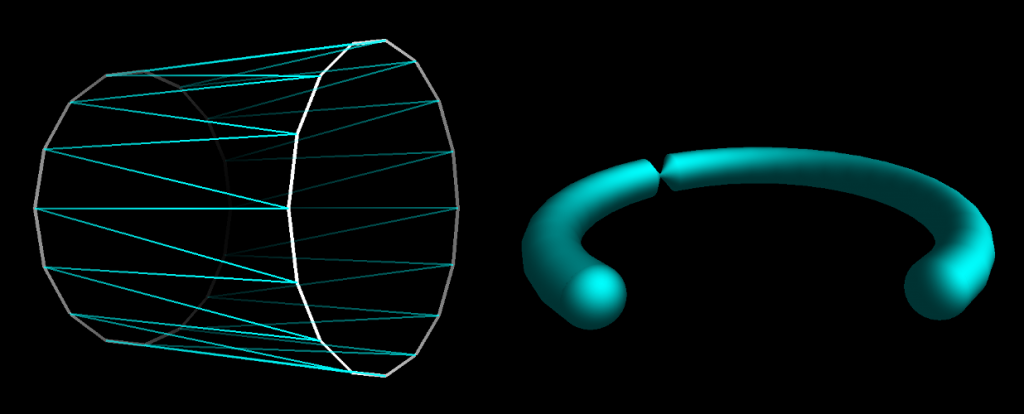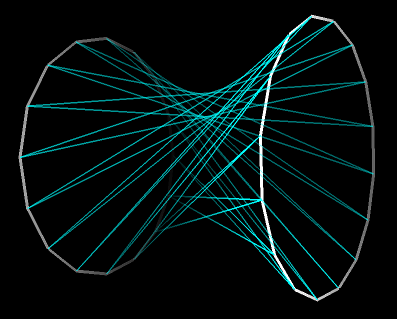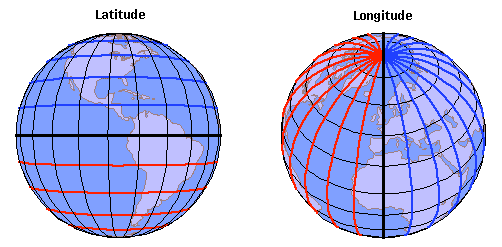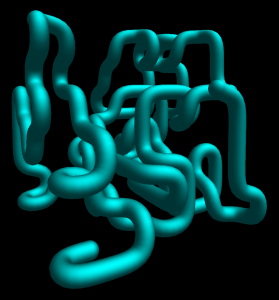# A Gentleman’s Orthonormal Basis Rotation

So you need to draw curved and bent pipe-tube-things.

You can make a straight section with two rings of points; just orient one ring like so, the other ring like so, and draw triangles between them. For a curve, just string a bunch of short straight pieces together.Almost but not quite. Why the hell is there that pinched part in the curved pipe?

The problem is that the orientation of the rings is not as simple as “normal to the pipe’s tangent”. But a ring is a circular-symmetrical thing, right, so rotation about the pipe’s tangent shouldn’t really matter. Right?

Wrong. These rings are not symmetric. For one thing they’re made up of finite points, so they aren’t even rings; they’re regular polygons. More importantly, the points in each aren’t interchangeable, they’re ordered. A “ring” has a first point, second point… and last point. The triangles drawn between the rings are drawn between corresponding points in those sequences. If those sequences don’t agree, you’ll get a hyperboloid instead of a cylinder. The pipe isn’t pinched, it’s twisted.To orient something in 2D you only need one angle, as there’s only one possible axis to rotate about in a plane. From one angle you can cook up the unique unit vector:
```vector.x = cos(angle) vector.y = sin(angle)```
.. and with comically simple math, a 90-degree rotation of it:
`vector(x,y).rotated90() == vector(-y,x)`

Those two vectors form an orthonormal basis in two dimensions, more specifically a rotation of the 2D standard basis. That one angle is like a coordinate around the circumference of a circle; it tells you a direction.

A third dimension complicates things.

To specify a direction in 3D you need two angles, just as you need two coordinate values to specify a point on a sphere.Converting two spherical coordinates into a unit vector in rectangular coordinates can be done in many ways, and the details aren’t important. However you come up with this handy little unit vector, you don’t have all the information you need. You’ve got a direction, but you don’t have a complete orientation. Imagine you stand outside and face directly skyward. An “up” vector specifies the direction you’re looking, but you may turn left and right while still looking “up”. There’s another axis of rotation to worry about. You need a third coordinate value.

Ultimately, you need an orthonormal basis, ideally without using too much trigonometry.

If you only care about setting the direction of one of the basis’s units i.e. the other two units’ orientations can be anything as long as they’re orthogonal, you don’t have a problem. Take your initial vector, swap/negate values to form any other vector, and the cross product between those two will give you some vector that’s orthogonal to your first. One more cross product between the first and second gives you the third orthogonal vector.

The simplest approach to the pipe problem is to generate such a basis from the tangent of the pipe at each segment. I tried this first, hoping that almost-identical initial vectors (the neighboring tangents around the curve) would yield almost-identical bases. We already know how that turned out.

Another way is to carry an orthonormal basis along the pipe as it’s drawn, incrementally adjusting it from section to section. Just set the one basis vector equal to the next tangent and use cross products to re-orthogonal-ize the other two in the basis without changing them too radically. This kinda works OK, but only if the change in orientation is small. This technique still introduces a little hyperboloid twisting, and it falls apart completely for 90-degree orientation changes.

The basic idea of carrying along a basis and incrementally rotating it is solid, we just need to do that rotation properly. The needed rotation comes from the relationship of the adjacent pipe tangents, and it can be represented by a unit vector orthogonal to both plus the angle between them. Call the adjacent tangents A and B; the rotation axis is simply the normalized cross product, and the angle can get gotten with `atan2` and the dot product:
```axis = A⨯B / |A⨯B| angle = atan2(|A⨯B|, A·B)```

This axis-angle rotation can then be applied to all three vectors in the basis with Rodrigues’ formula. This gets the job done with one call each to `atan2()`, `sin()`, and `cos()` per pipe section, which isn’t too bad, but it’s not optimal. We don’t actually care what that angle value is; all that matters is replicating the rotation from A to B. All we need is the sine and cosine of the angle formed by A and B, and those can be calculated with just a square root or two:
```divisor = sqrt(|A⨯B|^2 + (A·B)^2) sin = |A⨯B| / divisor cos = A·B / divisor```

There we have it, pipe-tube in all kinds of meandering curving and bending and doubling-back and no weird twists or pinches and no trigonometry functions necessary.This entry was posted in Uncategorized and tagged . Bookmark the permalink.